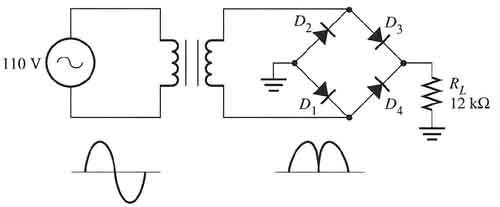# Full Wave Rectifier, Ripple Voltage?

Naptime

## Homework Statement

Full Wave Bridge Rectifier circuitBasically this circuit, but ZL is r//c not simply r as it is in this circuit (also, disregard any values... just the model is relevant)
Vs=6.5cost(2pi60t)
CL=1μF
RL=500Ω

I'm trying to find Vmax and Vripple.

## Homework Equations

Vmax = Vdc-2Von
Vr = (Vmax Tau) / (2RC)

Tau = 1/f <-- This must be wrong but it's the only Time constant equation I have.

## The Attempt at a Solution

Vmax = Vdc-2Von = 6.5 - 2(.5) = 5.5v
Tau = 1/f = 1/60 = .0166
Vr = (Vmax Tau) / (2RC) = 5.5 * Tau / (2(500)(1x10-6) = 91.6

My output graph (generated using SPICE) shows a sinusoid from around .2ish to 5ish Vo so clearly 91 Vripple is wrong. There should be a Vripple of about 5v to achieve this specific Vo. I'm 95% that my error is in the Tau calculation but I just can't find any info in my book/web about it.

Any help is appreciated, ty.

Homework Helper
Gold Member
First off 0.5 is a bit low for Vf of the diodes.

As for the ripple. I use..

Q=VC

differentiate to give

I=CdV/dt

rearrange to give

dV = Idt/C

So then need to find approx I and dt...

if V=5V and R = 500 Ohms

I=V/R
= 0.01Amps

dt is approx
1/(60*2) = 0.008s

so
dV = 0.01 * 0.008 / 1*10-6
= 80V

Except it can't be 80V as that's >> 5V.

In other words the capacitor is not big enough to remove any significant ripple. The ripple is therefore roughly same as the AC waveform. That appears to be what Spice is telling you.

Last edited:
Staff Emeritus
Hi Naptime. http://img96.imageshack.us/img96/5725/red5e5etimes5e5e45e5e25.gif [Broken]
CL=1μF
RL=500Ω

I'm trying to find Vmax and Vripple.
Check that you have the correct value for CL.

If CL is not large enough to produce small ripple, then the ripple will be large and the approximations involved in deriving Vr = (Vmax Tau) / (2RC) do not apply, hence that formula does not apply. CL of 1 μF is way too small to do anything useful here.

Last edited by a moderator:
Homework Helper
Gold Member
ps: The above method I used for calculating the ripple is only very approximate. It assumes the load is roughly constant current. That's a valid approximation when the ripple is small. If the ripple voltage is large it's not an accurate method.

However in this case the ripple appears so large and larger than the supply voltage that accuracy isn't that important.

Homework Helper
Gold Member
With a 500 ohm load you can effectively disregard the capacitor. Just assume the transformer is ideal and away you go. You won't get "constant load current" or anything near it with Cload = 1μF and Rload = 500Ω.

Different story, and much more complicated, if Rload = 12K as shown in the figure. There the rolloff is at ~ 13Hz and you're running 60Hz input voltage.

Naptime
OK I figured it out. Basically I was confusing Tau for T. Time constant and the period were the same thing in my calculations which threw everything off. This was basically a design where the output was designed poorly (on purpose so that we could learn to fix it). When Vo is ~ Vin the rectifier wasn't doing it's job. Basically the purpose is the flip the bottom half of the sinusoid to "mirror" the positive side and to do so the filter needed to be above a specific range (using the Vr equation in the first post, recalculate for C). If anyone needs me to fully rework this let me know, but with the help of those who responded and my TA I was able to redesign the circuit given the criteria. Ty.

Homework Helper
Gold Member
OK I figured it out. Basically I was confusing Tau for T. Time constant and the period were the same thing in my calculations which threw everything off. This was basically a design where the output was designed poorly (on purpose so that we could learn to fix it). When Vo is ~ Vin the rectifier wasn't doing it's job. Basically the purpose is the flip the bottom half of the sinusoid to "mirror" the positive side and to do so the filter needed to be above a specific range (using the Vr equation in the first post, recalculate for C). If anyone needs me to fully rework this let me know, but with the help of those who responded and my TA I was able to redesign the circuit given the criteria. Ty.

Good job. have a nice nap, Ty!